# Introduction

We saw in Chapter 6 that a linear tearing mode is unstable when the (real dimensionless) tearing stability index,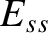(see Section 3.8), is positive, and is stable otherwise. In the linear regime, an unstable tearing mode grows exponentially in time on a timescale that is intermediate between the short hydromagnetic time,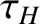[see Equation (5.43)], and the much longer resistive diffusion time,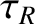[see Equation (5.49)]. The mode reconnects magnetic flux at the so-called rational surface to produce a helical magnetic island chain. (See Section 5.16.) The rational surface is the magnetic flux-surface at which the tearing mode resonates with the equilibrium magnetic field (i.e., at which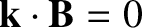, where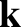is the wavevector of the mode, and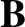the equilibrium magnetic field). The tearing mode also rotates in the laboratory frame at the angular frequency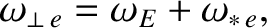(9.1)

where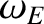and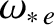are the E-cross-B and electron diamagnetic frequencies, respectively, at the rational surface. [See Equations (5.21), (5.29), (5.44), and (5.45).] In fact, the previous equation implies that the tearing mode co-rotates with the electron fluid at the rational surface.

We saw in Section 5.16 that linear tearing mode theory breaks down as soon as the radial width of the magnetic island chain exceeds the linear layer width, and must be replaced by nonlinear theory. The aim of this chapter is to employ the analysis of Chapter 8 to determine the nonlinear time evolution of an unstable tearing mode.